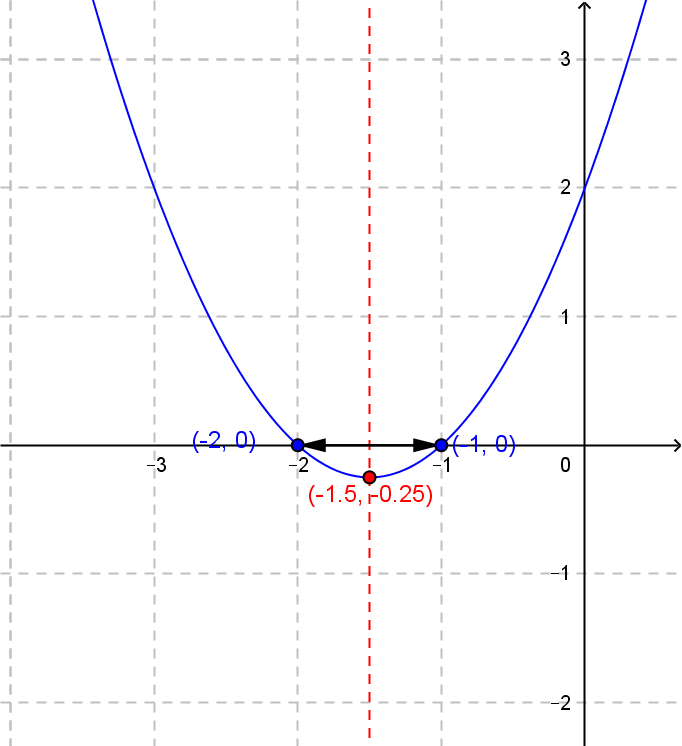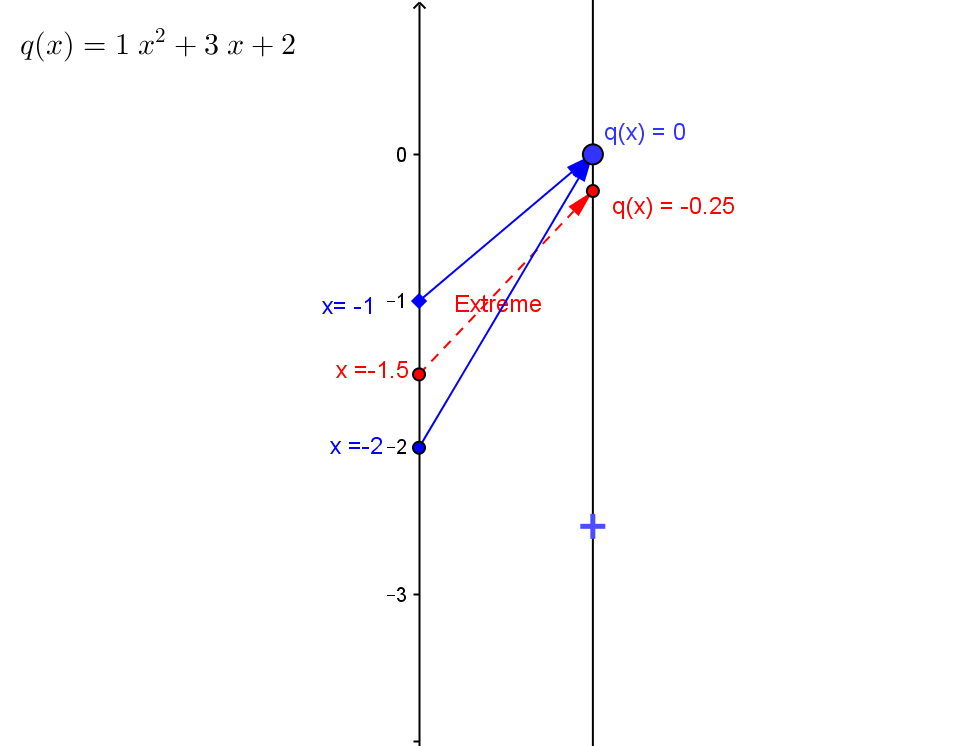Example  QEQ.2.  Suppose $5x^2 + 4x + 5 = 4x^2 + x + 3$. Find $x$.
Solution: This is solved by solving the related equation $Ax^2 + Bx+C= 0$ where $A = A_1 - A_2 = 5-4 =1$, $B = B_1 - B_2 =4 -1 = 3$, and $C = C_1 - C_2= 5-3 = 2$.
The related equation is $x^2 +3x +2 = 0$.
Since $A \ne 0$, the equation can be solved with $x = \frac {-B \pm \sqrt{ B^2 - 4 A C}} {2A}$  where $A=1$, $B=3$ and $C = 2$.
So the solution is $x = \frac {-3 \pm \sqrt{ 3^2 - 4 *1* 2}} {2*1} = \frac {-3 \pm \sqrt{ 9 - 8}} {2} = \frac {-3 \pm 1} {2}$.
So the solutions are $x = -1$ and $x=-2$.

Comment: We can consider the expression on the left hand side of the equation as a function of $x$ giving $f(x) = x^2 +3x +2$ . Now the problem can be restated: to find a $x$ where $f(x) = 0$. This problem and its solution can be visualized both on the graph and the mapping diagram for the function $f$.
 For the graph of $f$: Find $y=0$ on the Y axis , then find the point(s) on the graph of $f$ with second coordinate $0$, determine it's first coordinates, $-1$ and $-2$, and those are the desired values for $x$. For the mapping diagram of $f$: Find $y=0$ on the target axis , then find point(s) $x$ on the source axis with the function arrow pointing to $0$.To do this, look for the point(s) on the $X$ axis, the line $y=0$, where the axis intersects the graph of $f$ To do this, find the extreme point of $f$ on the mapping diagram, $x = -\frac B {2A} = -\frac 3 2$. Move symmetrically above and below that value by $\pm \frac 1 2$, which are the desired values for $x$.